login  home  contents  what's new  discussion  bug reports help  links  subscribe  changes  refresh  edit

The following example appeared in Maxima mailing list (Maxima could not do it). Here we solve it using FriCAS. First we form system of equations:

fricas
(1) -> -- Form system of equations
eqn1 := a1*x1^5 + a2*x2^5 + a3*x3^5 = 1 / 6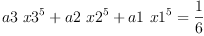(1)
Type: Equation(Polynomial(Fraction(Integer)))
fricas
eqn2 := a1*x1^4 + a2*x2^4 + a3*x3^4 = 1 / 5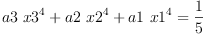(2)
Type: Equation(Polynomial(Fraction(Integer)))
fricas
eqn3 := a1*x1^3 + a2*x2^3 + a3*x3^3 = 1 / 4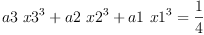(3)
Type: Equation(Polynomial(Fraction(Integer)))
fricas
eqn4 := a1*x1^2 + a2*x2^2 + a3*x3^2 = 1 / 3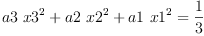(4)
Type: Equation(Polynomial(Fraction(Integer)))
fricas
eqn5 := a1*x1 + a2*x2 + a3*x3 = 1 / 2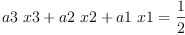(5)
Type: Equation(Polynomial(Fraction(Integer)))
fricas
eqn6 := a1 + a2 + a3 = 1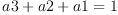(6)
Type: Equation(Polynomial(Integer))
fricas
eqns := [eqn1, eqn2, eqn3, eqn4, eqn5, eqn6];
Type: List(Equation(Polynomial(Fraction(Integer))))

Now we solve it

fricas
solve(eqns, [a1, a2, a3, x1, x2, x3])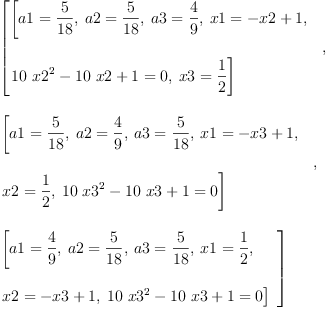(7)
Type: List(List(Equation(Fraction(Polynomial(Integer)))))

The solution above is not fully explicit, in first solotion we need to solve quadratic equation in x2 (so this solution really represents two solutions, one for each solution to the quadratic equation). Similarly, second and third solution contain quadratic equation in x3. Usualy such form of solution is convenient for further computations. But one can also get more explicit version of solution using 'radicalSolve':

fricas
radicalSolve(eqns, [a1, a2, a3, x1, x2, x3])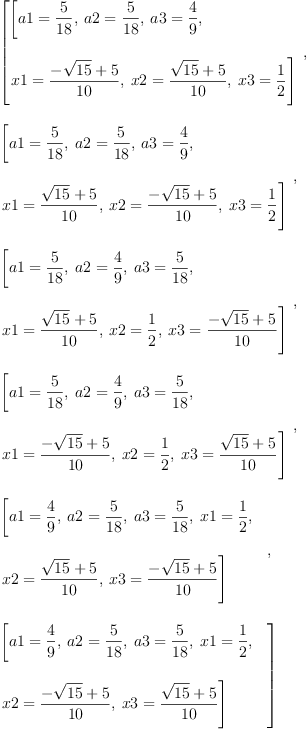(8)
Type: List(List(Equation(Expression(Integer))))

Note: not all equations have solutions in radicals and FriCAS can find solutions in radicals only in relatively simple cases. But system above has simple solution in radicals and FriCAS can find it.

 Subject:   Be Bold !! ( 14 subscribers )